﻿ 忆阻器新型非线性窗口函数的伏安特性研究 Research on the Voltammetric Characteristics of a New NonlinearWindow Function of Memristor

Biophysics
Vol.05 No.04(2017), Article ID:23150,8 pages
10.12677/BIPHY.2017.54004

Research on the Voltammetric Characteristics of a New Nonlinear Window Function of Memristor

Shuoqiao Xie, Jiqian Zhang, Fei Xu, Jiansheng Zhang, Shoufang Huang, Tingting Fang

College of Physics and Electronic Information, Anhui Normal University, Wuhu Anhui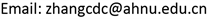Received: Dec. 4th, 2017; accepted: Dec. 18th, 2017; published: Dec. 25th, 2017ABSTRACT

In this paper, based on the memristor model with our new window function, the regulation of voltammetric characteristics was studied by adjusting the related parameters of the memristor model. Simulation results show that, firstly, the existing window functions have some shortcomings: It is easy to appear the terminal state problem, and the flexibility of regulation needs to be improved. Secondly, to simulate the evolution of the voltammetric characteristics, the internal parameters describing the characteristics of the window function is selected as the control variables. Furthermore, to study the dynamical behavior of memristor the external excitation signal is introduced and the frequency of the external excitation signal is adjusted. The results show that our new window function not only can effectively solve the shortcomings of the existing window function, but also has a good ability to identify the stimulus signals with different frequencies. These results will help us to further study the nonlinear characteristics of memristor, and have theoretical significance and guidance for further understanding of the mechanism of memristor and the development of electronic devices with specific functions.

Keywords:Memristor, Window Function, Voltammetric Characteristics, Terminal State Problem1. 引言

2. 忆阻器模型描述

$\text{d}\phi =M\text{d}q$ (1)

2008年惠普实验室提出了一个基于TiO2的双极忆阻器：在两个Pt电极中间加上有高阻特性的纯净TiO2和低阻特性的掺杂TiO2−x组成的TiO2薄膜，此时忆阻器总电阻为掺杂区域电阻加上非掺杂区域电阻之和的可变电阻  ，忆阻值M(ω)可以表示为：

$M\left(\omega \right)=\frac{\omega }{D}{M}_{on}+\left(1-\frac{\omega }{D}\right){M}_{off}$ (2)

TiO2−x缺少氧原子，携带一定的电荷。在两个Pt电极之间的电场的作用下，带电杂质TiO2−x开始发生偏移，TiO2和TiO2−x的分界面随之迁移。分界面的移动速度取决于电流强度。用状态变量ω表示完全

 。D为忆阻器薄膜的宽度，Mon和Moff分别为完全掺杂以及完全无掺杂时忆阻器阻值。

$\frac{\text{d}\omega }{\text{d}t}=\frac{{\mu }_{V}{M}_{on}}{D}i\left(t\right)g\left(x\right)$ (3)

$x=\frac{\omega }{D}$ (4)

$\frac{\text{d}x}{\text{d}t}=\frac{{\mu }_{V}{M}_{on}}{{D}^{2}}i\left(t\right)g\left(x\right)$ (5)

x用来描述掺杂区与忆阻器宽度的比值， ${\mu }_{V}$ 为离子迁移速率。i(t)表示通过忆阻器的电流，引入激励电压 $V\left(t\right)=M\left(q\left(t\right)\right)i\left(t\right)$$g\left(x\right)$ 为边界迁移的窗口函数，描述TiO2和TiO2−x分界面迁移的非线性掺杂动力学行为，当 $g\left(x\right)=1$ 时，掺杂边界为线性迁移。

3. 结果与讨论

3.1. 非线性窗口函数

$g\left(x\right)=x-{x}^{2}$ (6)

$g\left(x\right)=1-{\left(2x-1\right)}^{2p}$ (7)

$\begin{array}{l}g\left(x\right)=1-{\left[x-\mathrm{sgn}\left(-i\right)\right]}^{2p}\\ \left\{\begin{array}{l}\mathrm{sgn}\left(i\right)=1\begin{array}{ccc}& & i\ge 0\end{array}\\ \mathrm{sgn}\left(i\right)=0\begin{array}{ccc}& & i<0\end{array}\end{array}\end{array}$ (8)

$g\left(x\right)=q×\left(1-{\left[{\left(x-0.5\right)}^{2}+0.75\right]}^{p}\right)$ (9)

$g\left(x\right)=q×{\mathrm{sin}}^{\frac{1}{p}}\left(\text{π}x\right)$ (10)

3.2. 伏安特性曲线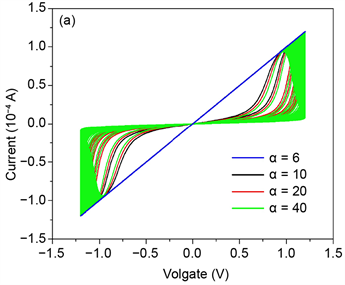Figure 1. Current-voltage curves with different α values.Figure 2. Current-voltage curves with different x values.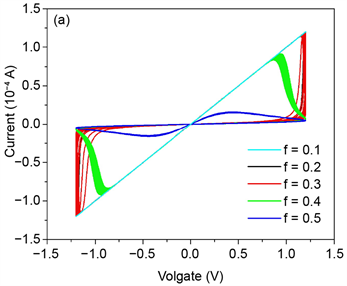Figure 3. Current-voltage curves with different f values.

4. 总结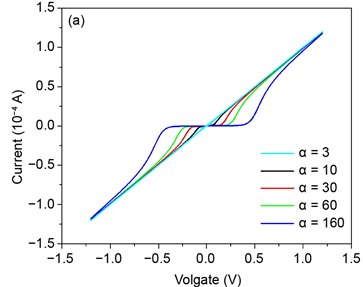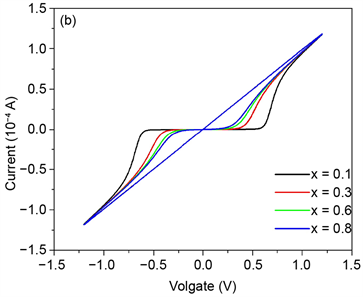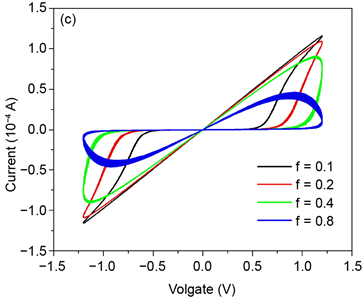Figure 4. Current-voltage curves with different parameters values. (a) x = 0.25, f = 0.05 Hz, α = 3, 10, 30, 60, 160. Current-voltage curves with different α values. (b) α = 160, f = 0.05 Hz, x = 0.1, 0.5, 0.6, 0.8. Current-voltage curves with different x values. (c) x = 0.25, α = 160, f = 0.1, 0.2, 0.4, 0.8. Current-voltage curves with different f values

Research on the Voltammetric Characteristics of a New NonlinearWindow Function of Memristor[J]. 生物物理学, 2017, 05(04): 25-32. http://dx.doi.org/10.12677/BIPHY.2017.54004

1. 1. Chua, L.O. (1971) Memristor—The Missing Circuit Element. IEEE Transactions on Circuit Theory, 18, 507-519. https://doi.org/10.1109/TCT.1971.1083337

2. 2. Itoh, M. and Chua, L.O. (2014) Dynamics of Memristor Circuits. International Journal of Bifurcation and Chaos, 24, 1430015. https://doi.org/10.1142/S0218127414300158

3. 3. Itoh, M. and Chua, L.O. (2008) Memristor Oscillators. International Journal of Bifurcation and Chaos, 18, 3183-3206. https://doi.org/10.1142/S0218127408022354

4. 4. 王伟, 曾以成, 孙睿婷. 含三个忆阻器的六阶混沌电路研究[J]. 物理学报, 2017, 66(4): 25-35.

5. 5. 包伯成, 刘中, 许建平. 忆阻混沌振荡器的动力学分析[J]. 物理学报, 2010, 59(6): 3785-3793.

6. 6. Bao, B.C., Xu, J.P. and Liu, Z. (2010) Initial State Dependent Dynamical Behaviors in Memristor Based Chaotic Circuit. Chinese Physics Letters, 27, 5.

7. 7. Borghetti, J., Snider, G.S., Kuekes, P.J., Yang, J.J., Stewart, D.R. and Williams, R.S. (2010) “Memristive” Switches Enable “Stateful” Logic Operations via Material Implication. Nature, 464, 873-876. https://doi.org/10.1038/nature08940

8. 8. Zhu, X., Yang, X.J., Wu, C.Q., Xiao, N., Wu, J.J. and Yi, X. (2013) Performing Stateful Logic on Memristor Memory. IEEE Transactions on Circuits and Systems II: Express Briefs, 60, 682-686. https://doi.org/10.1109/TCSII.2013.2273837

9. 9. Yu, H., Ni, L. and Huang, H. (2017) Distributed In-Memory Computing on Binary Memristor-Crossbar for Machine Learning, Advances in Memristors, In: Vaidyanathan, S. and Volos, C., Eds., Memristive Devices and Systems, Springer Intern. Publishing, 275-304.

10. 10. Ohno, T., Hasegawa, T., Tsuruoka, T., Terabe, K., Gimzewski, J.K., Aono, M., et al. (2011) Short-Term Plasticity and Long-Term Potentiation Mimicked in Single Inorganic Synapses. Nature Materials, 10, 591-595. https://doi.org/10.1038/nmat3054

11. 11. Cabaret, T., Fillaud, L., Jousselme, B., et al. (2015) Electro-Grafted Organic Memristors: Properties and Prospects for Artificial Neural Networks Based on STDP. IEEE International Conference on Nanotechnology, 499-504.

12. 12. Yang, J., Wang, L.d., Wang, Y. and Guo, T.T. (2017) A Novel Memristive Hopfield Neural Network with Application in Associative Memory. Neurocomputing, 227, 142-148. https://doi.org/10.1016/j.neucom.2016.07.065

13. 13. Pershin, Y. and Ventra, M.D. (2010) Experimental Demonstration of Associative Memory with Memristive Neural Networks. Neural Networks, 23, 881-886. https://doi.org/10.1016/j.neunet.2010.05.001

14. 14. Carbajal, J.P., Dambre, J. and Hermans, M., et al. (2014) Memristor Models for Machine Learning. Neural Computation, 27, 1-23.

15. 15. Ni, L., Huang, H. and Yu, H. (2016) A Memristor Network with Coupled Oscillator and Crossbar towards L2-Norm Based Machine Learning. Nanoscale Architectures (NANOARCH), IEEE/ACM International Symposium on Nanoscale Architectures. IEEE, Beijing, 179-184.

16. 16. Versace, M. and Chandler, B. (2010) The Brain of a New Machine. IEEE Spectrum, 47, 30-37. https://doi.org/10.1109/MSPEC.2010.5644776

17. 17. 冯广, 段书凯, 王丽丹. 双极性脉冲忆阻桥电子突触神经网络及图像处理[J]. 中国科学: 信息科学, 2017, 47(3): 385-400.

18. 18. 贾林楠, 黄安平, 郑晓虎, 肖志松, 王玫. 界面效应调制忆阻器研究进展[J]. 物理学报, 2012, 61(21): 217306-217306.

19. 19. 田晓波. 忆阻器电路特性与应用研究[D]: [硕士学位论文]. 长沙: 国防科学技术大学, 2009.

20. 20. Joglekar, Y.N. and Wolf, S.J. (2009) The Elusive Memristor: Properties of Basic Electrical Circuits. European Journal of Physics, 30, 661-675. https://doi.org/10.1088/0143-0807/30/4/001

21. 21. Biolek, Z., Biolek, D. and Biolkova, V. (2009) SPICE Model of Memristor with Nonlinear Dopant Drift. Radioengineering, 18, 210-214.

22. 22. Prodromakis, T., Peh, B.P., Papavassiliou, C., et al. (2011) A Versatile Memristor Model with Nonlinear Dopant Kinetics. IEEE Transactions on Electron Devices, 58, 3099-3105. https://doi.org/10.1109/TED.2011.2158004

23. 23. Indiveri, G., Linares-Barranco, B., Legenstein, R., et al. (2013) Integration of Nanoscale Memristor Synapses in Neuromorphic Computing Architectures. Nanotechnology, 24, 384010-384032. https://doi.org/10.1088/0957-4484/24/38/384010